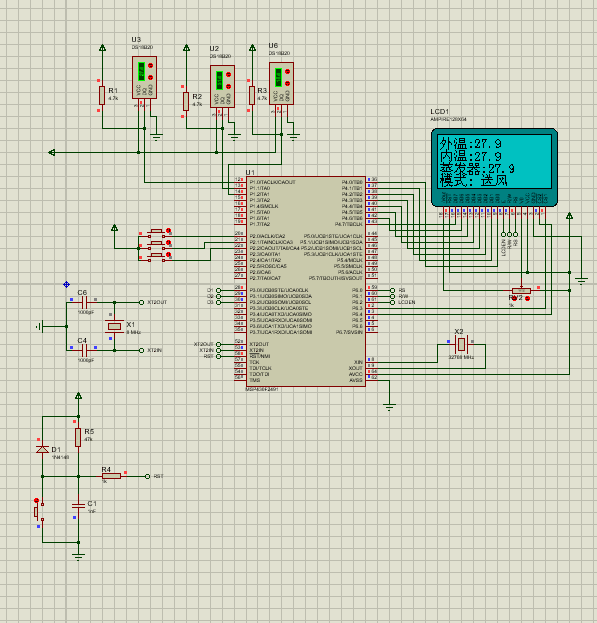proteus 仿真 ds18b20 温度始终显示27.9 然后我的按钮也失灵，求能教我怎么做我尝试了并行三个温度传感器 但是温度一直显示不对，然后试了下只编程一个 也是27.9不知道为什么 然后我自己做的按钮，编程好像也有点问题，求会做的教我，追加悬赏80

/*******************************************

********************************************/
uchar DS18B20_Reset(void)
{
uchar Error=0;
P1DIR = 0xff;

_DINT();
P1OUT = 0x00;

DelayNus(500);

P1OUT = 0xff;

DelayNus(55);

P1DIR = 0x00;

_NOP();

if(P1IN == 0x00)

{
Error = 1; //初始化成功
}
else
{
Error = 0; //初始化失败
}
P1DIR = 0xff; //设置为输出，并MCU给出高电平
P1OUT = 0xff;
_EINT();
DelayNus(400);
return Error;
}

//总线读取一个数据位
{
uchar DQ_S=0;
P1OUT = 0x00; //8个DQ 线全部设置为低电平
DelayNus(6);
P1OUT = 0xff;
DelayNus(8);
P1DIR = 0x00; //准备读取
_NOP(); //延时待总线准备好数据
DQ_S = P1IN; //一次性读取8条DQ线的数据状态
DelayNus(45);
P1DIR = 0xff;
P1OUT = 0xff; //恢复端口电平
DelayNus(10); //延时
return DQ_S; //返回读取的值

}

//写数据
void DS18B20_WriteByte(uchar Com)
{
uchar i;
_DINT();
for(i=0;i {
P1DIR = 0xff;
P1OUT = 0x00;
DelayNus(6);
if (Com&0x01)
{
P1OUT = 0xff;
}
else
{
P1OUT = 0x00;
}
Com = Com>>1;
DelayNus(50);
P1OUT = 0xff;
DelayNus(10);
}
_EINT();
}
// 录入两位数据
{
uint i;
for(i=0;i<16;i++)
{
}
}

//启动温度转换
void DS18B20_Conver(void)
{
DS18B20_WriteByte(0xcc);
DS18B20_WriteByte(0x44);
}

//读取温度值
{
uchar i;
char j,k;
do
{
i = DS18B20_Reset();
}
while(i);
DS18B20_Conver();
DelayNus(60000);
do
{
i = DS18B20_Reset();
}
while(i);
DS18B20_WriteByte(0xcc);
DS18B20_WriteByte(0xbe);
for(j=16;j>0;j--)
{
for(k=0;k<3;k++)
{
uiData[k] = uiData[k]<<1;
}
}
}

//将十一位二进制数转换成六位整形数据

void Disp_Numb(uint temper)
{
uchar i;
for(i = 0;i < 6;i++) dN[i] = 0;

``````if(temper & BIT0)
{
dN = 5;
dN = 2;
dN = 6;
}
if(temper&BIT1)
{
dN += 5;
dN += 2;
dN += 1;
}
if(temper & BIT2)
{
dN += 5;
dN += 2;
if(dN >= 10)
{
dN -= 10;
dN += 1;
}
}
if(temper&BIT3)
{
dN += 5;
}
if(temper & BIT4)
{
dN += 1;
}
if(temper & BIT5)
{
dN += 2;
}
if(temper & BIT6)
{
dN += 4;
}
if(temper & BIT7)
{
dN += 8;
if(dN >= 10)
{
dN -= 10;
dN += 1;
}
}
if(temper & BIT8)
{
dN += 6;
dN += 1;
if(dN >= 10)
{
dN -= 10;
dN += 1;
}
}
if(temper & BIT9)
{
dN += 2;
dN += 3;
if(dN >= 10)
{
dN -= 10;
dN += 1;
}
}
if(temper & BITA)
{
dN += 4;
dN += 6;
if(dN >= 10)
{
dN -= 10;
dN += 1;
}
if(dN >= 10)
{
dN -= 10;
}
}
``````

}

//显示当前温度

void Disp_TEM(void)
{
char Bai,Shi,Ge; //定义变量
Disp_Numb(Cur_TMP1);
Bai=dN;
Shi=dN;
Ge =dN;
Disp_EN(1,6,table1[Bai]);
Disp_EN(1,7,table1[Shi]);
Disp_EN(1,8,table1);
Disp_EN(1,9,table1[Ge]);
DelayNus(100);
Disp_Numb(Cur_TMP2);
Bai=dN;
Shi=dN;
Ge =dN;
Disp_EN(2,6,table1[Bai]);
Disp_EN(2,7,table1[Shi]);
Disp_EN(2,8,table1);
Disp_EN(2,9,table1[Ge]);
DelayNus(100);
Disp_Numb(Cur_TMP3);
Bai=dN;
Shi=dN;
Ge =dN;
Disp_EN(3,8,table1[Bai]);
Disp_EN(3,9,table1[Shi]);
Disp_EN(3,10,table1);
Disp_EN(3,11,table1[Ge]);
DelayNus(100);
}

//按键检测

uchar Keyscan()
{
uchar temp,key;
P2DIR=0x00;
temp=P2IN;
if (temp==0x01)
{
DelayNus(10);
if (temp==0x01) key=1;
}
if (temp==0x02)
{
DelayNus(10);
if (temp==0x02) key=2;
}
if (temp==0x04)
{
DelayNus(10);
if (temp==0x03) key=3;
}
else
{
DelayNus(10);
}
return key;
}

//主程序
int main(void)
{
WDTCTL=WDTPW+WDTHOLD;

``````BCSCTL1 &= ~XT2OFF;
_EINT();//打开全局中断
TACTL |= TASSEL_2 + ID_3;
Ini_Lcd();

while(1)
{
GDWZ();
Cur_TMP1=uiData;
Cur_TMP2=uiData;
Cur_TMP3=uiData;
Disp_TEM();
key_number=Keyscan();
if (key_number==1)
{
if(Cur_TMP1<=10)
{
P3DIR = 0x05;
P3OUT = BIT0+BIT2;
Disp_HZ(4,4,table2);
Disp_HZ(4,5,table2);

}
if(Cur_TMP1>10&&Cur_TMP1<20)
{
P3DIR = 0x01;
P3OUT = BIT0;
Disp_HZ(4,4,table2);
Disp_HZ(4,5,table2);
}
else
{
P3DIR = 0x03;
P3OUT = BIT0+BIT1;
Disp_HZ(4,4,table2);
Disp_HZ(4,5,table2);
}
}
if(key_number==2)
{
if(Cur_TMP1<=20)
{
P3DIR = 0x05;
P3OUT = BIT0+BIT2;
Disp_HZ(4,4,table2);
Disp_HZ(4,5,table2);
}
if(Cur_TMP1>20&&Cur_TMP1<30)
{
P3DIR = 0x01;
P3OUT = BIT0;
Disp_HZ(4,4,table2);
Disp_HZ(4,5,table2);
}
else
{
P3DIR = 0x03;
P3OUT = BIT0+BIT1;
Disp_HZ(4,4,table2);
Disp_HZ(4,5,table2);
}
}
if (key_number==3)
{
if(Cur_TMP1<=30)
{
P3DIR = 0x05;
P3OUT = BIT0+BIT2;
Disp_HZ(4,4,table2);
Disp_HZ(4,5,table2);
}
if(Cur_TMP1>30&&Cur_TMP1<40)
{
P3DIR = 0x01;
P3OUT = BIT0;
Disp_HZ(4,4,table2);
Disp_HZ(4,5,table2);
}
else
{
P3DIR = 0x03;
P3OUT = BIT0+BIT1;
Disp_HZ(4,4,table2);
Disp_HZ(4,5,table2);
}
}
else
{
P3DIR = 0x01;
P3OUT = BIT0;
Disp_HZ(4,4,table2);
Disp_HZ(4,5,table2);
}
Delay_Nms(1000);
Clear_LCD();
}
``````

}

4个回答wuxiaotian_1 最后算是完成了，我也不管这个问题有没有成功。。。wuxiaotian_1 我的程序我检查了一下找不出问题 我想看看自己错在哪

/*一下是 .H文件*/
#ifndef __DS18B20_H
#define __DS18B20_H
#include
#include
#define uchar unsigned char
#define uint unsigned int

void delay_us(uint t);//us级延迟函数
void DS18B20_reset();//单总线通信的初始化
void DS18B20_write_byte(uchar dat);//位写入时序
uint Read_ID_temperature(uchar x);//寻找ID，温度读取子函数 （ 多个DS18B20时使用 ）

#endif

/*一下是.C文件*/

#include

sbit DS18B20=P2^0;
bit flag;

void delay_us(uint t)//us级延迟函数
{
while(t--);
}
void DS18B20_reset()//单总线通信的初始化
{
DS18B20=1;
delay_us(5);//约66us
DS18B20=0;
delay_us(80);//约700+us
DS18B20=1;
delay_us(14);//约150us
if(DS18B20==0)
flag=1;//表示18B20存在
else
flag=0;//表示18B20不存在
delay_us(20);//约200+us
}
{
bit dat; //定义 位的变量
DS18B20=0;
nop();
nop();
DS18B20=1;
nop();
dat=DS18B20;
delay_us(10);//约100us
return dat;
}
{
uchar i,j,k;
for(i=0;i {
k=(j>1);
}
return k;
}
void DS18B20_write_byte(uchar dat)//位写入时序
{
uchar i;
for(i=0;i {
DS18B20=0;
_nop_();
DS18B20=dat&0x01;
delay_us(6);//约70us
DS18B20=1;
dat=dat>>1;//右移一位

}
delay_us(6);
}
//{
// uint temp;//定义一个16位变量
// uchar a=0,b=0;
// float TEMP=0;
// DS18B20_reset();//DS18B20 初始化
// DS18B20_write_byte(0xCC); // 跳过读序号列号的操作
// DS18B20_write_byte(0x44); // 启动温度转换
// DS18B20_reset();//DS18B20 初始化
// DS18B20_write_byte(0xcc);//发送ROM操作命令0xcc，跳过Rom命令
// DS18B20_write_byte(0xbe);//发送温度读取命令(读暂存器)0xbe
//

//
// temp=b;
// temp=temp<<8;//左移8位
// temp=temp|a;//就此，temp的值便是温度的16位
// TEMP=temp*0.0625;//[算法],得出实际温度。将二进制数转化为温度的值
// temp=TEMP*10+0.5;//方便显示，放大10倍;进行4舍5入，去掉小数
// return (temp);
//}

uchar code DS18B20_A_ID={0x28,0x15,0xc4,0x40,0x05,0x00,0x00,0x46}; //短引脚18B20
uchar code DS18B20_B_ID={0x28,0x62,0x0B,0xD3,0x06,0x00,0x00,0x9B}; //长引脚18B20

uint Read_ID_temperature(uchar x)//寻找ID，温度读取子函数 （ 多个DS18B20时使用 ）
{
uchar i;
uint temp;//定义一个16位变量
uchar a=0,b=0;
float TEMP=0;
DS18B20_reset();//复位18B20
DS18B20_write_byte(0x55); // 寻找ID命令
for(i=0;i<8;i++)//写入64位ROM代码 （要读取温度的ID号）
{
if(x==1)
{
DS18B20_write_byte(DS18B20_A_ID[i]);
}
if(x==2)
{
DS18B20_write_byte(DS18B20_B_ID[i]);
}
}

``````DS18B20_write_byte(0x44); // 启动温度转换
DS18B20_reset();//复位18B20
DS18B20_write_byte(0x55); // 寻找ID命令
for(i=0;i<8;i++)//写入64位ROM代码 （要读取温度的ID号）
{
if(x==1)
{
DS18B20_write_byte(DS18B20_A_ID[i]);
}
if(x==2)
{
DS18B20_write_byte(DS18B20_B_ID[i]);
}
}
DS18B20_write_byte(0xbe);//发送温度读取命令(读暂存器)0xbe

temp=b;
temp=temp<<8;//左移8位
temp=temp|a;//就此，temp的值便是温度的16位
TEMP=temp*0.0625;//[算法],得出实际温度。将二进制数转化为温度的值
temp=TEMP*10+0.5;//方便显示，放大10倍;进行4舍5入，去掉小数
return (temp);
``````

}wuxiaotian_1 我是并行多个io口 每个io口一个温度传感器 不需要读取ID号 读取太麻烦了wuxiaotian_1 就是数据交互失败，可能时序有问题，现在就失败的做上去了，，，然后解释说是软件问题Proteus仿真——18B20+数码管显示温度（含源码）
Proteus仿真18B20+数码管显示温度
ATMEGA16读取DS18B20温度，1602显示，并用proteus仿真，成功。
ATMEGA16单片机读取DS18B20的温度数据，用lcd1602显示，并用proteus实现仿真。 实现温度的实时读取，程序中设定温度的上下限，当温度超限时报警，用led小灯进行 指示。    共同学习 q  ：1425636507

ds18b20温度传感器最简系统 程序+proteus仿真图

keil uvsion4文件目录 89c52rc单片机 DS18B20芯片 晶振12M intrins.h reg52.h stdio.h三个文件是keil自带的 delay.h #ifndef __DELAY_H__ #define __DELAY_H__ /*------------------------------------------------  uS延时函数，含有输

DS1302 与微处理器进行数据交换时，首先由微处理器向电路发送命令字节，命令字节最高位Write Protect(D7)必须为逻辑1，如果D7=0，则禁止写DS1302，即写保护；D6=0，指定时钟数据，D6=1，指定RAM数据；D5～D1指定输入或输出的特定寄存器；最低位LSB(D0)为逻辑0，指定写操作(输入)， D0=1，指定读操作(输出)。 在DS1302的时钟日历或RAM进行

DS18B20之Proteus仿真使用

51单片机DS18B20程序及proteus仿真

<br />#include<reg51.h><br />#include<stdlib.h><br />#include<stdio.h>//头文件<br />#define uchar unsigned char<br />#define uint unsigned int<br />sbit DQ=P2^6;//ds18b20与单片机连接口<br />sbit PWM=P2^5;//控制信号的输出<br />unsigned char code str[]={"my name is zhb "};<b

proteus仿真代码，基于单片机的实时温度日历显示

DS18B20显示温度并报警
DS18B20显示温度并报警 2016-07-24 花花雪 跟单片机谈恋爱 目录： 引言 1理论     1.1DS18B20原理     1.2系统原理图 2程序 ~~~~~~~~~~~~~~~~~~~~~~~~~~~~~~~~~~~~~~~~~~~~~~~~~~~ 引言     这个系统想要实现的功能： （1

//温度测试 DS18B20 //精确计算，含一位小数， 液晶显示，用的是12864 改程序好用
DS18B20和AT89C51实现的温度采集器的仿真电路和源程序代码

DS18B20采集的温度实时显示在lcd液晶屏上

STM32单片机(11) DS18B20温度传感器实验

STC15系列读取DS18B20温度传感器串口显示代码

1. 中断介绍       简单的来说，当CPU在正常运行的时候内部或外部发生某一件事要求CPU立刻去处理，这时CPU就会中断当前的工作去处理请求执行的事情，处理之后再返回到原来中止的地方接着工作。如图4-1。（4-1）      单片机的中断系统通过四个相关的特殊功能寄存器TCON、SCON、IE和IP来进行管理，因此用户可以用软件对每个中断的开和关以及优先级的控制作用。如图4-2。（4-...

proteus仿真实例 DS18B20
proteus仿真实例 DS18B20proteus仿真实例 DS18B20proteus仿真实例 DS18B20proteus仿真实例 DS18B20proteus仿真实例 DS18B20
LCD12864（里面包括源代码和使用Proteus仿真的程序）（不需要硬件也可以仿真）
LCD12864（里面包括源代码和使用Proteus仿真的程序）（不需要硬件也可以仿真）

DS18B20程序，精确到小数点后两位
/********************************************************************************                               DS18B20 测温程序    硬件：AT89c52     (1)单线ds18b20接 P2.2    (2)七段数码管接P0口    (3)使用外部电源给ds18b2
DS18B20温度传感器利用LCD显示室内温度
#include #include "./delay/delay.h" #include "./lcd/lcd.h" #include "intrins.h" #include "stdio.h" sbit DS = P3^2; sbit led = P3^3; bit ack; void ds18b20_init() { DS = 0; delay_us(200); dela

（Proteus模拟）基于51单片机的波形发生器

#include #include sbit DQ=P1^4;//温度采集 sbit latch1=P2^2;//段锁存 sbit latch2=P2^3;//位锁存 unsigned char code dofly_DuanMa={0x3f,0x06,0x5b,0x4f,0x66,0x6d,0x7d,0x07,0x7f,0x6f};//显示段码0~9 unsigned cha

4*4矩阵键盘+1602显示的proteus仿真
4*4矩阵键盘+1602液晶显示的proteus仿真实验
ds18b20温度采集仿真